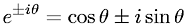Equations > Trigonometry > Trigonometric Identities > Euler's Formula

### Euler's FormulaLatex Code:

MathML Code:

 ${e}^{±i\theta }=\mathrm{cos}\theta ±i\mathrm{sin}\theta$

MathType 5.0: